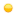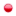###Author Topic: Regarding delta funciton for the interaction.  (Read 2325 times)

#### yeongjingwak

• Newbie
•• Posts: 3##### Regarding delta funciton for the interaction.
HI. I'm currently delta function as an interaction.

I found that the numerical data is not identical with the analytic one.

For that reason, I wonder how the program approximates delta function.

1. How does the program approximate the Delta function?

2. Does the method have any difference between the analytic delta function?

3. How can I reduce the difference between the analytic delta function and the delta function in the MCTDH-X program?

#### mctdhb

• Newbie
•• Posts: 15##### Re: Regarding delta funciton for the interaction.
Hi there,

It's of importance for what specific problem you ask this question.

For contact interactions, we usually directly evaluate the W_sl integrals analytically and work with this.
There's thus no difference with the analytical delta distribution. In more than one spatial dimension, however, issues do arise with contact potentials.

Then, in >1D, one needs either renormalization or just to consider a short-ranged interaction with the same scattering properties (see for instance: Phys. Rev. A 87, 033631 (2013) ).

Regards,
Axel